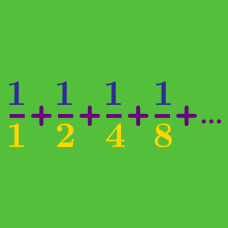Calculus

# Visual Patterns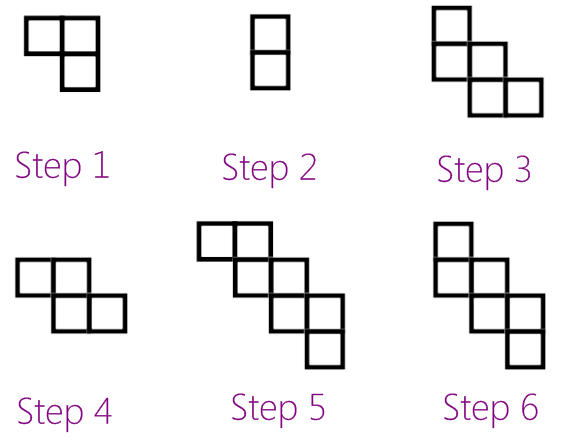How many squares will be in step 99?

(HINT: Look at just the odd-numbered steps.)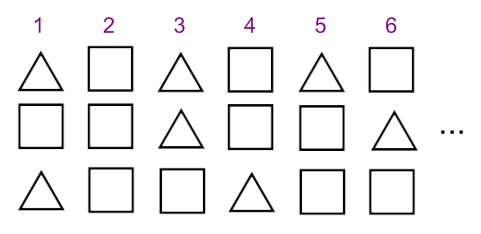How many triangles will there be in column 100?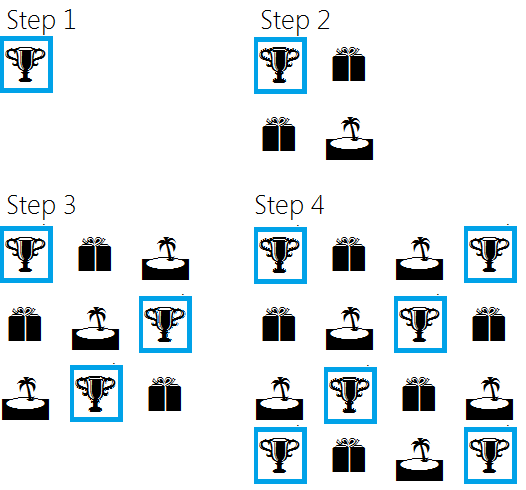At step 40, how many trophies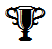will there be in the 7th row?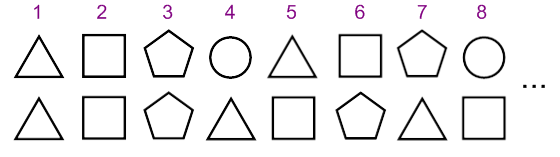The first column is the first time that two triangles appear as a pair.

Which column is the tenth time that two triangles appear as a pair?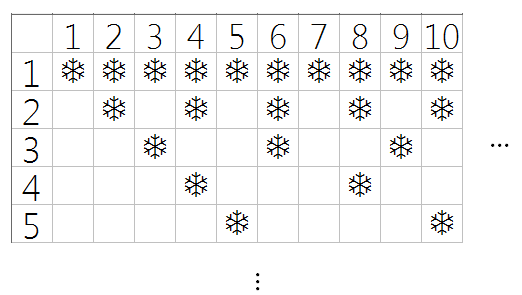In the first row, every column is filled; in the 2nd row, every 2nd column has a snowflake; in the 3rd row, every 3rd column has a snowflake; in the 4th row, every 4th column has a snowflake.

The pattern continues in both the columns and rows.

Which of these numbers will have exactly two snowflakes in its column?

×